• Slides: 22Warm-Up Exercises 1. Evaluate ANSWER 2. Evaluate ANSWER 1 3. 2 1 8 1 – 2. 4 16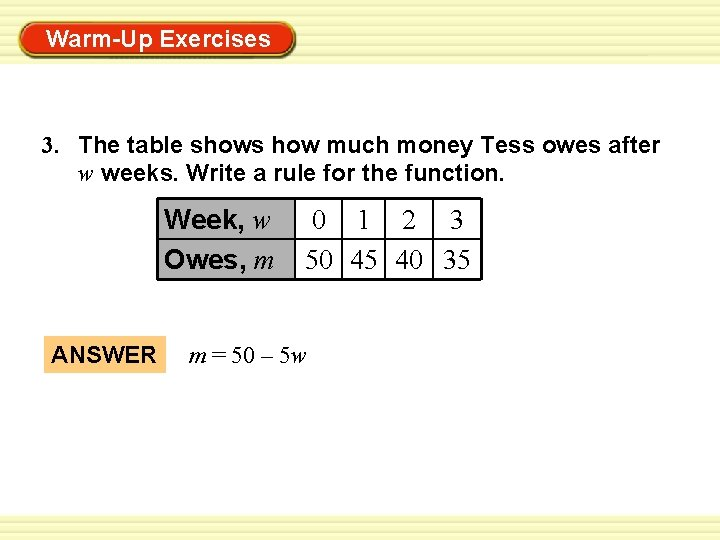Warm-Up Exercises 3. The table shows how much money Tess owes after w weeks. Write a rule for the function. Week, w Owes, m ANSWER 0 1 2 3 50 45 40 35 m = 50 – 5 w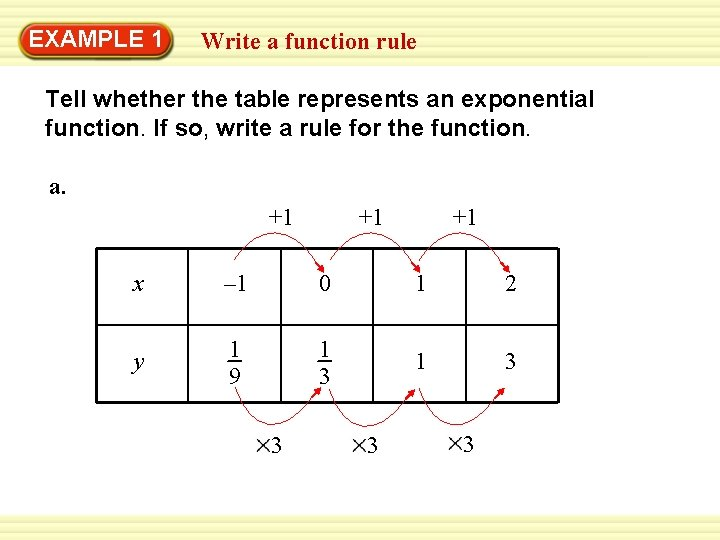EXAMPLE Warm-Up 1 Exercises Write a function rule Tell whether the table represents an exponential function. If so, write a rule for the function. a. +1 +1 +1 x – 1 0 1 2 y 1 9 1 3 3 3 3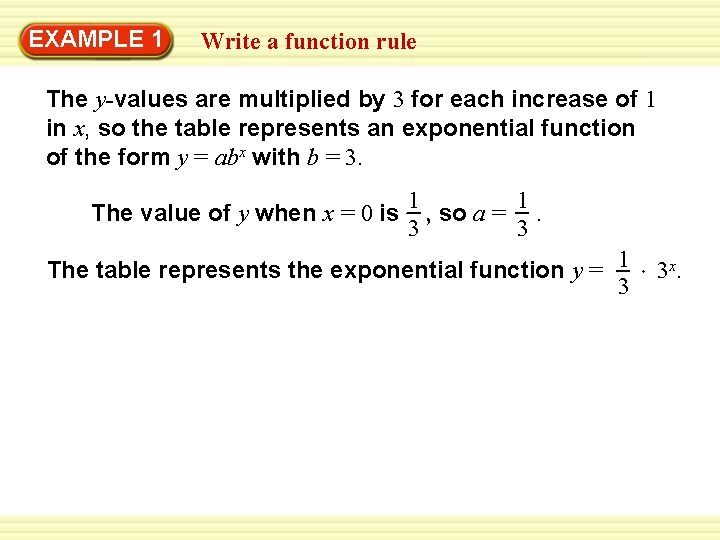EXAMPLE Warm-Up 1 Exercises Write a function rule The y-values are multiplied by 3 for each increase of 1 in x, so the table represents an exponential function of the form y = abx with b = 3. The value of y when x = 0 is 1 1 , so a =. 3 3 The table represents the exponential function y = 1 3 3 x.EXAMPLE Warm-Up 1 Exercises Write a function rule b. +1 x y – 1 4 +1 +1 0 1 2 1 1 4 1 16 1 1 4 4 1 The y-values are multiplied by for each increase 4 of 1 in x, so the table represents an exponential function of the form y = abx with b = 1. 4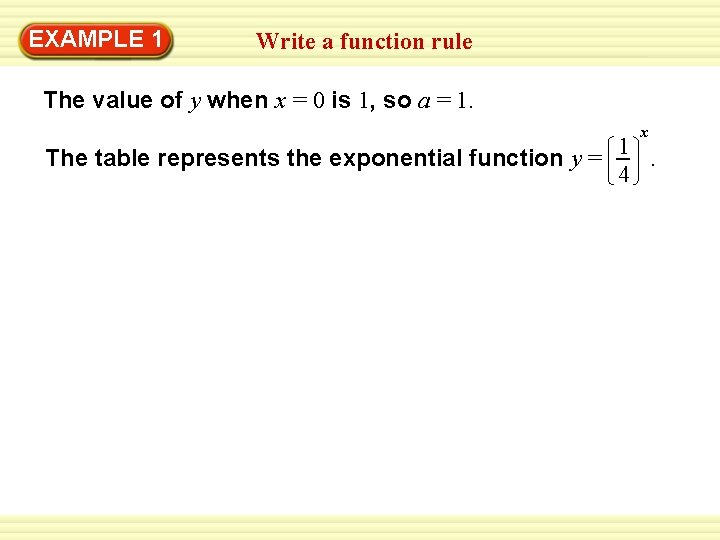EXAMPLE Warm-Up 1 Exercises Write a function rule The value of y when x = 0 is 1, so a = 1. x The table represents the exponential function y = 1. 4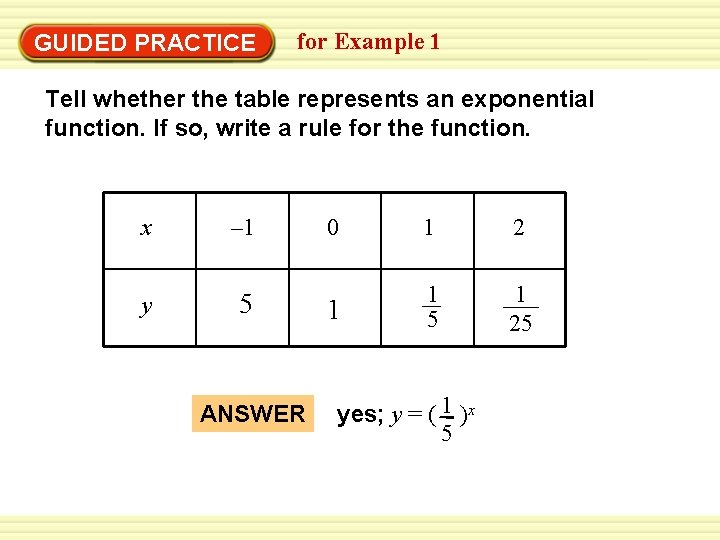Warm-Up Exercises GUIDED PRACTICE for Example 1 Tell whether the table represents an exponential function. If so, write a rule for the function. x – 1 y 5 ANSWER 0 1 2 1 1 5 1 25 yes; y = ( 1 )x 5Warm-Up 2 Exercises EXAMPLE Graph an exponential function 1 Graph the function y = 2 range. x and identify its domain and SOLUTION STEP 1 Make a table of values. The domain is all real numbers. x – 2 – 1 0 1 2 y 4 2 1 1 2 1 4Warm-Up 2 Exercises EXAMPLE Graph an exponential function STEP 2 Plot the points. STEP 3 Draw a smooth curve through the points. From either the table or the graph, you can see the range is all positive real numbers.Warm-Up 3 Exercises EXAMPLE Compare graphs of exponential functions Graph the functions y = 3 1 1 x and y = − 2 3 1 x 2 1 x Compare each graph with the graph of y = 2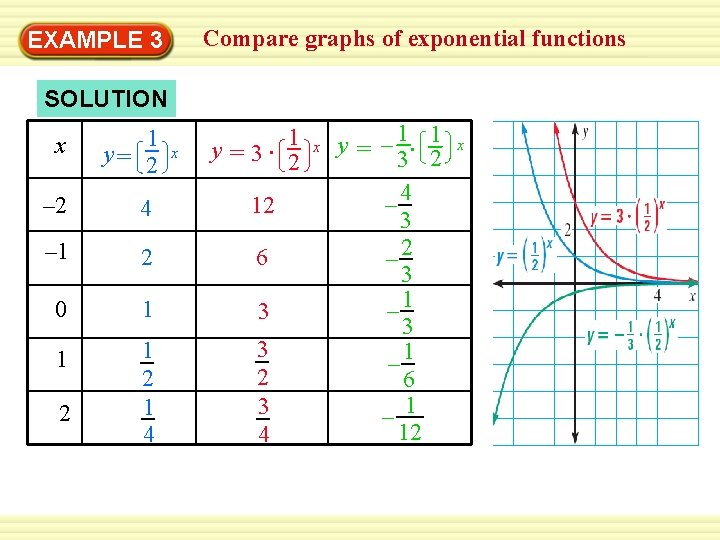Compare graphs of exponential functions Warm-Up 3 Exercises EXAMPLE SOLUTION 1 y =3∙ 2 x 1 y= 2 – 2 4 12 – 1 2 6 0 1 3 1 1 2 1 4 3 2 3 4 2 x x y = – 1∙ 1 3 2 – 4 3 – 2 3 – 1 6 – 1 12 xWarm-Up 3 Exercises EXAMPLE Compare graphs of exponential functions 1 x Because the y-values for y = 3 2 are 3 times the 1 x corresponding y-values for y = , the graph of 2 1 x y=3 is a vertical stretch of the graph of y =. 2 2 1 1 x are – 1 times the Because the y-values for y = – 3 2 3 1 x corresponding y-values for y = 2 , the graph of 1 1 x y = – 3 2 is a vertical shrink with reflection in the 1 x x-axis of the graph of y = 2.Warm-Up Exercises GUIDED PRACTICE for Examples 2 and 3 2. Graph the function y = (0. 4)x and identify its domain and range. ANSWER Domain: all real numbers Range: all positive real numbers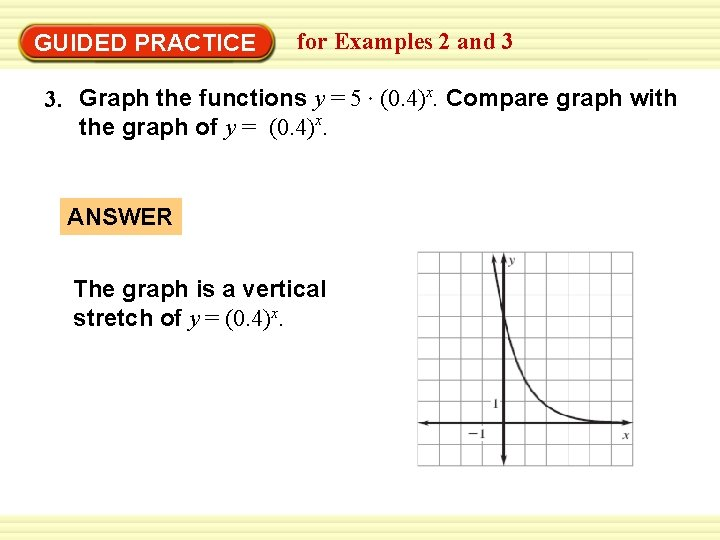Warm-Up Exercises GUIDED PRACTICE for Examples 2 and 3 3. Graph the functions y = 5 (0. 4)x. Compare graph with the graph of y = (0. 4)x. ANSWER The graph is a vertical stretch of y = (0. 4)x.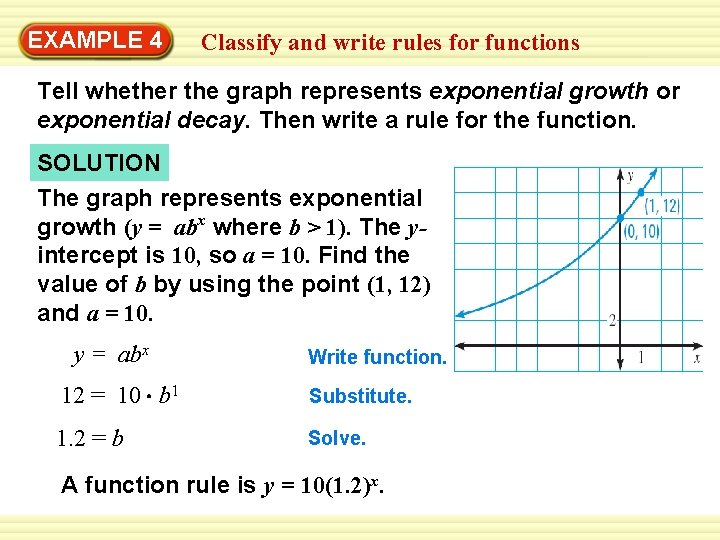Warm-Up 4 Exercises EXAMPLE Classify and write rules for functions Tell whether the graph represents exponential growth or exponential decay. Then write a rule for the function. SOLUTION The graph represents exponential growth (y = abx where b > 1). The yintercept is 10, so a = 10. Find the value of b by using the point (1, 12) and a = 10. y = abx Write function. 12 = 10 b 1 Substitute. 1. 2 = b Solve. A function rule is y = 10(1. 2)x.Warm-Up 4 Exercises EXAMPLE Classify and write rules for functions Tell whether the graph represents exponential growth or exponential decay. Then write a rule for the function. The graph represents exponential decay (y = abx where 0 < b < 1). The y-intercept is 8, so a = 8. Find the value of b by using the point (1, 4) and a = 8. y = abx Write function. 4 = 8 b 1 Substitute. 0. 5 = b Solve. A function rule is y = 8(0. 5)x.Warm-Up Exercises GUIDED PRACTICE for Example 4 4. The graph of an exponential function passes through the points (0, 10) and (1, 8). Graph the function. Tell whether the graph represents exponential growth or exponential decay. Write a rule for the function. ANSWER exponential decay; y = 10 (0. 8)x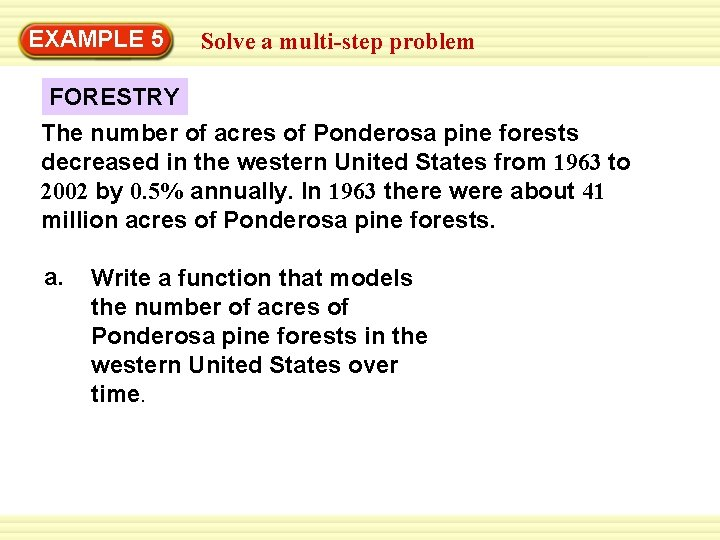EXAMPLE Warm-Up 5 Exercises Solve a multi-step problem FORESTRY The number of acres of Ponderosa pine forests decreased in the western United States from 1963 to 2002 by 0. 5% annually. In 1963 there were about 41 million acres of Ponderosa pine forests. a. Write a function that models the number of acres of Ponderosa pine forests in the western United States over time.EXAMPLE Warm-Up 5 Exercises Solve a multi-step problem b. To the nearest tenth, about how many million acres of Ponderosa pine forests were there in 2002? SOLUTION a. Let P be the number of acres (in millions), and let t be the time (in years) since 1963. The initial value is 41, and the decay rate is 0. 005. P = a(1 – r)t Write exponential decay model. = 41(1 – 0. 005)t Substitute 41 for a and 0. 005 for r. = 41(0. 995)t Simplify.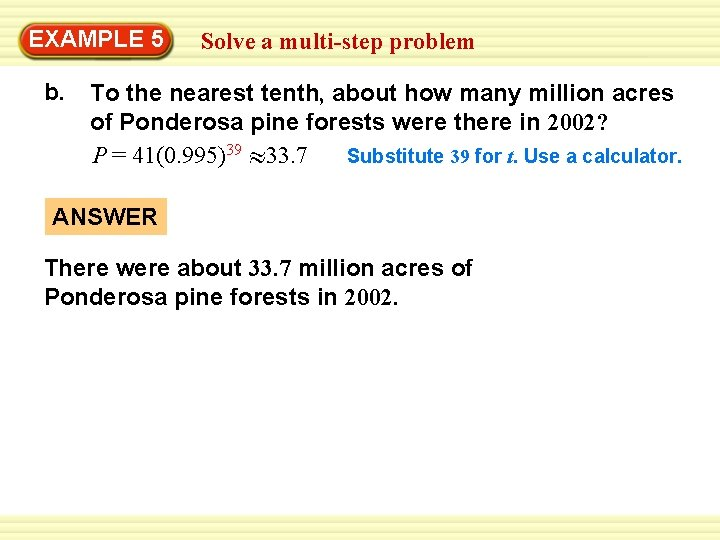EXAMPLE Warm-Up 5 Exercises Solve a multi-step problem b. To the nearest tenth, about how many million acres of Ponderosa pine forests were there in 2002? P = 41(0. 995)39 33. 7 Substitute 39 for t. Use a calculator. ANSWER There were about 33. 7 million acres of Ponderosa pine forests in 2002.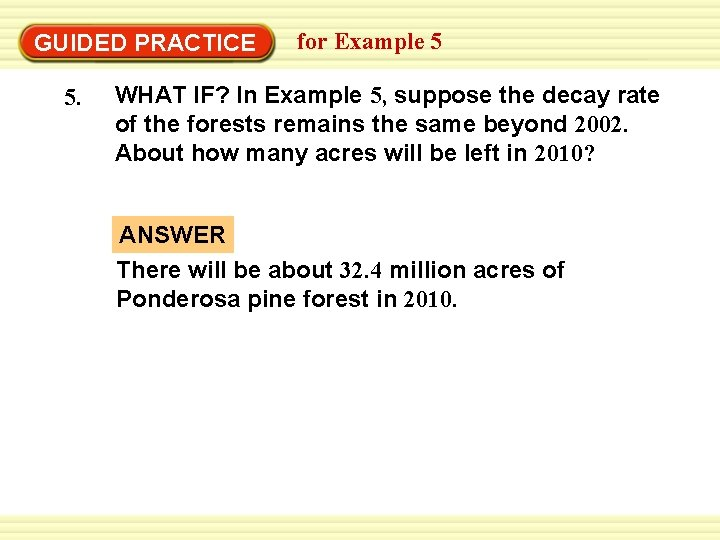Warm-Up Exercises GUIDED PRACTICE 5. for Example 5 WHAT IF? In Example 5, suppose the decay rate of the forests remains the same beyond 2002. About how many acres will be left in 2010? ANSWER There will be about 32. 4 million acres of Ponderosa pine forest in 2010.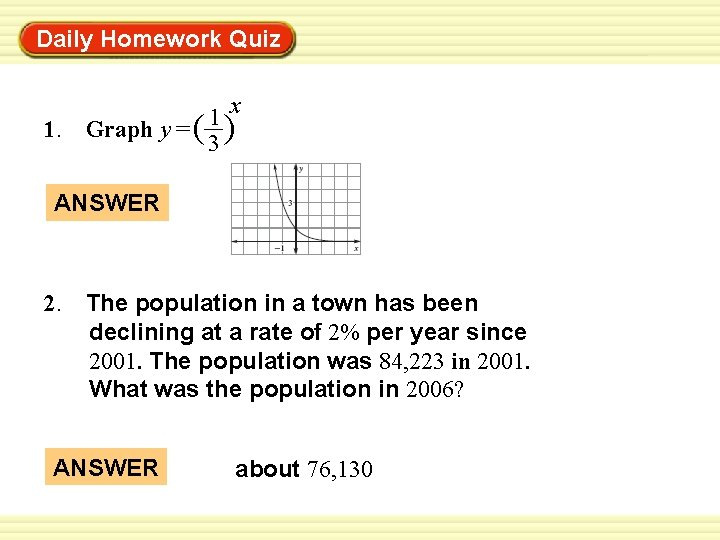Daily Homework Quiz Warm-Up Exercises x 1 1. Graph y = ( ) 3 ANSWER 2. The population in a town has been declining at a rate of 2% per year since 2001. The population was 84, 223 in 2001. What was the population in 2006? ANSWER about 76, 130